Lacuna

For lacunae in function theory see e.g. Hadamard theorem on gaps; Fabry theorem on gaps; Lacunary power series.

For lacunae in geometry see Group of motions; Lacunary space.

A lacuna in the theory of partial differential equations is a subdomainof the intersection of the interior of the characteristic cone of a linear hyperbolic system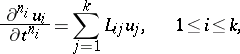(1)

with vertex at the point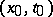and a plane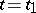. This subdomain is defined by the following property: small sufficiently smooth changes of the initial data insidedo not affect the value of the solutionat the point. In (1) it is assumed that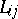is a linear differential operator of orderand that the order of the differentiations in it with respect todoes not exceed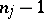. A "change inside" means a change in some domain that together with its boundary lies in.

For the wave equation(2)

the solutionof the Cauchy problem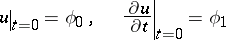(3)

at the point,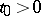, is completely determined by the values of the functions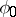and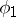on the spherefor oddand in the ballfor evenand, hence the domain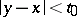in the planeis a lacuna for equation (2) for odd. For evenand forequation (2) has no lacuna. This agrees with the Huygens principle for solutions of the wave equation.

A perturbation of the initial data (3) in a small spherical neighbourhood of the pointleads to a spherical wave with centre at this point, which for oddhas outward and inward facing fronts. For the remaining values ofthe inward facing front of this wave is "diffused" ; this phenomenon is called diffusion of waves. Diffusion of waves is characteristic of all linear second-order hyperbolic equations if the numberof space variables is even (see ). The analogous question forwas studied in , where a class of second-order hyperbolic equations was described for which diffusion of waves is absent. The equations of this class are closely connected with the wave equation. For general hyperbolic systems (1) a relation "locally" has been found between the existence of a lacuna for the system (1) and the analogous question for the corresponding system with constant coefficients (see ). For the latter systems necessary and sufficient conditions of algebraic character have been obtained that ensure the presence of a lacuna.